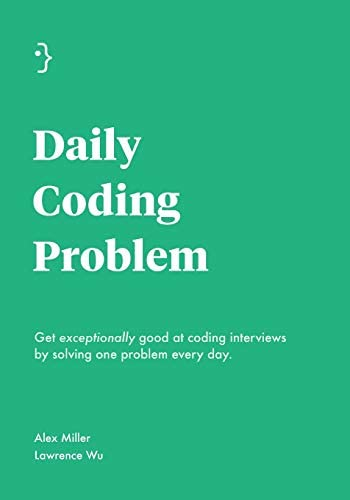# Tricks vs implementation in coding interviews

Marton Trencseni - Sat 22 May 2021 - Interviewing

Recently I bought the book Daily Coding Problem. I bought it because:

• I find these sorts of problems intellectually pleasing
• it's a good way to keep myself sharp as a programmer
• perhaps I can re-purpose some of the problems for Data Science interviews.The problems and solutions in the book reminded me of my own interviewing experiences, as a candidate: I get nervous in interview situations and don't do well on coding interviews. Doctors call this the white coat effect:

The alerting reaction to the physician's visit is known to induce a blood pressure rise termed "white coat effect." This phenomenon has often been associated with a clinical condition characterized by a persistently high blood pressure in the doctor's office and a persistently normal blood pressure at other times.

In other words, the measurement ("how well can this person program") result is below the actual truth. Let's look at an example:

Given an array of numbers, find the maximum sum of any contiguous subarray of the array.

I think any candidate for a programming job can be expected to code up the $O(n^3)$ brute force solution, but I'm not sure I would figure out the more efficient $O(n)$ solution known as Kadane's algorithm, with my blood pressure at 160-170, instead of my normal 135, in 3-5 minutes flat:

def max_sum(arr):
max_sum = float('-inf')
cur_sum = 0
for x in arr:
cur_sum = max(0, cur_sum + x)
max_sum = max(max_sum, cur_sum)
return max_sum


So as a hiring manager, I think a lot about how to make hiring loops less painful and more fair. It occured to me that potentially a better interviewing approach would be to:

1. Ask the candidate to figure out the naive / brute force solution on their own, and code it up.
2. Then, tell them verbally what the optimal solution is, and have them code it up.

Why?

1. Removing the step of the candidate having to think up tricky solutions would remove significant pressure.
2. It still tests the most important think: ability to implement without errors and write clean code.

The example above is not a good example, because Kadane's algorithm, once written down, is so simple, it's hard to "tell it" without effectively giving the candidate the solution itself. A better example is:

Given an array of numbers, find the bounds of the smallest window that must be sorted in order for the whole array to be sorted. For example, for the input [3, 7, 5, 6, 9], return [1, 3].

So:

1. Candidate gives the naive / brute force solution: copy and sort the array, then compare with the original. Time complexity is $O(n log(n) )$.
2. Tell the candidate that: Traverse the array and note whether the element is less than the maximum up to that point. If it is, this element would have to be part of the sorting window, since it's out of order compared to that previous maximum. Same in the reverse direction. Time complexity is $O(n)$.

Implementing the algorithm is still not trivial in an interview setting:

def min_window(arr):
left, right = None, None
max_seen, min_seen = float('-inf'), float('inf')

for i in range(len(arr)):
max_seen = max(max_seen, arr[i])
if arr[i] < max_seen:
right = i

for i in range(n - 1, -1, -1):
min_seen = min(min_seen, arr[i])
if arr[i] > min_seen:
left = i

return left, right


This seems like a better interviewing approach, because in a work setting, thinking up tricky solutions in 3-5 minutes is not a requirement. Usually, there are days or weeks for that. But implementing an idea, once the idea is there, should be straightforward for a good programmer.